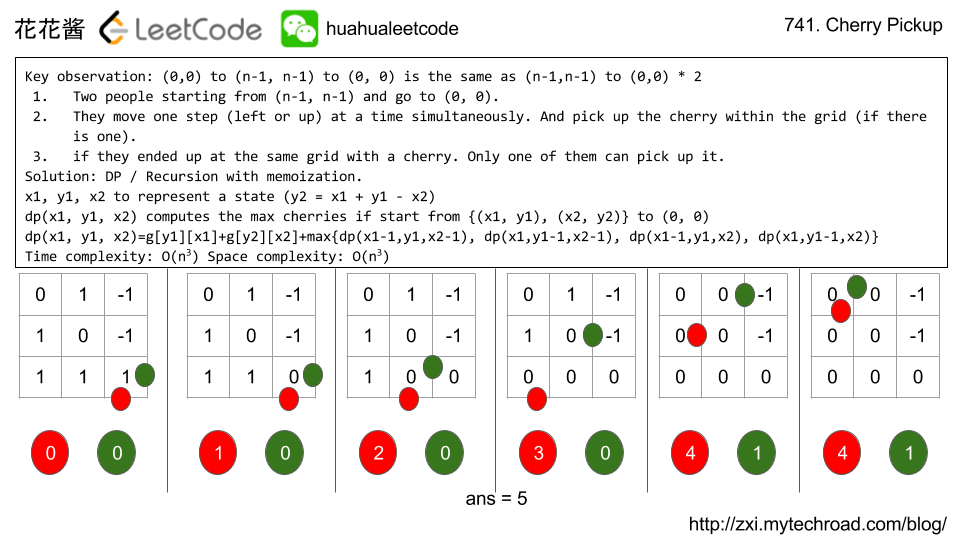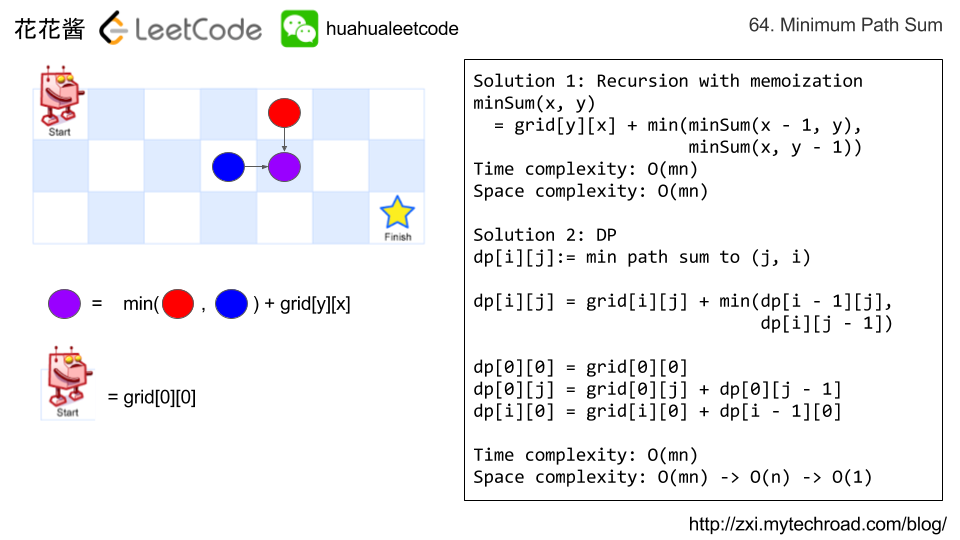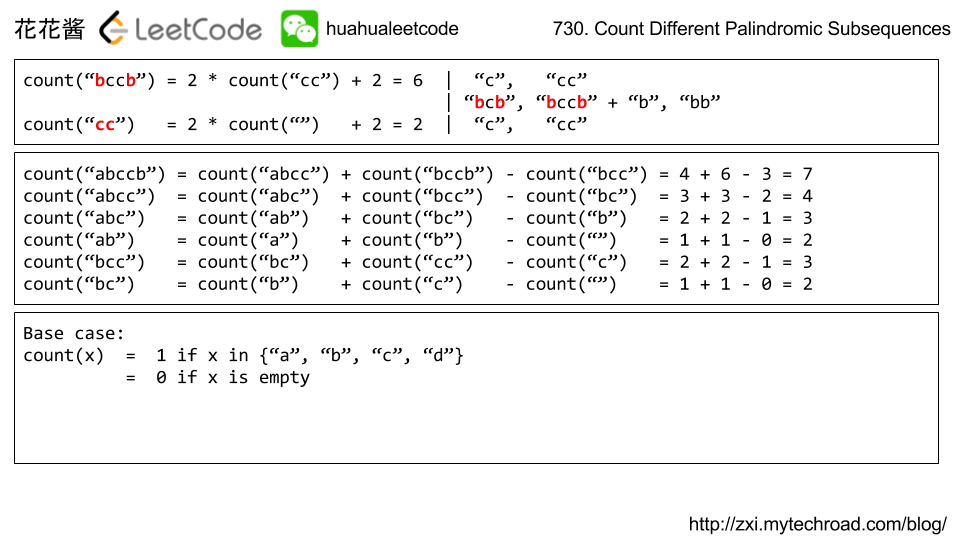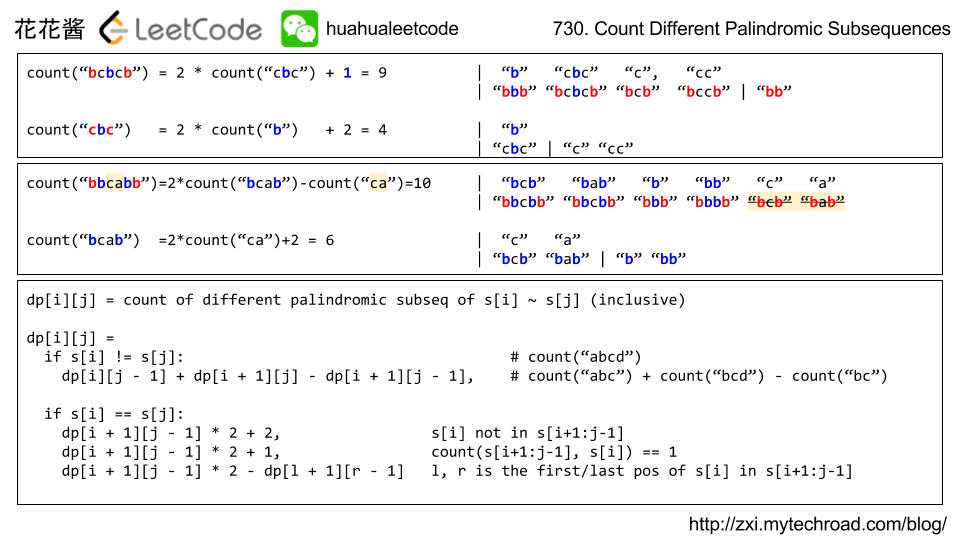# Posts tagged as “dp”

Problem:

In a N x N grid representing a field of cherries, each cell is one of three possible integers.

• 0 means the cell is empty, so you can pass through;
• 1 means the cell contains a cherry, that you can pick up and pass through;
• -1 means the cell contains a thorn that blocks your way.

Your task is to collect maximum number of cherries possible by following the rules below:

• Starting at the position (0, 0) and reaching (N-1, N-1) by moving right or down through valid path cells (cells with value 0 or 1);
• After reaching (N-1, N-1), returning to (0, 0) by moving left or up through valid path cells;
• When passing through a path cell containing a cherry, you pick it up and the cell becomes an empty cell (0);
• If there is no valid path between (0, 0) and (N-1, N-1), then no cherries can be collected.

Example 1:

Note:

• grid is an N by N 2D array, with 1 <= N <= 50.
• Each grid[i][j] is an integer in the set {-1, 0, 1}.
• It is guaranteed that grid and grid[N-1][N-1] are not -1.

Idea:

DP

Key observation: (0,0) to (n-1, n-1) to (0, 0) is the same as (n-1,n-1) to (0,0) twice

1. Two people starting from (n-1, n-1) and go to (0, 0).
2. They move one step (left or up) at a time simultaneously. And pick up the cherry within the grid (if there is one).
3. if they ended up at the same grid with a cherry. Only one of them can pick up it.

Solution: DP / Recursion with memoization.

x1, y1, x2 to represent a state y2 can be computed: y2 = x1 + y1 – x2

dp(x1, y1, x2) computes the max cherries if start from {(x1, y1), (x2, y2)} to (0, 0), which is a recursive function.

Since two people move independently, there are 4 subproblems: (left, left), (left, up), (up, left), (left, up). Finally, we have:

dp(x1, y1, x2)= g[y1][x1] + g[y2][x2] + max{dp(x1-1,y1,x2-1), dp(x1,y1-1,x2-1), dp(x1-1,y1,x2), dp(x1,y1-1,x2)}

Time complexity: O(n^3)

Space complexity: O(n^3)# Solution: DP

Time complexity: O(n^3)

Space complexity: O(n^3)

## Java

Related Problems:

Problem:

Given a m x n grid filled with non-negative numbers, find a path from top left to bottom right which minimizes the sum of all numbers along its path.

Note: You can only move either down or right at any point in time.

Example 1:

Given the above grid map, return 7. Because the path 1→3→1→1→1 minimizes the sum.

Idea:

DPSolution 1:

C++ / Recursion with memoization

Time complexity: O(mn)

Space complexity: O(mn)

Solution 2:

C++ / DP

Time complexity: O(mn)

Space complexity: O(1)

Related Problems:

Problem:

Find the contiguous subarray within an array (containing at least one number) which has the largest sum.

For example, given the array [-2,1,-3,4,-1,2,1,-5,4],
the contiguous subarray [4,-1,2,1] has the largest sum = 6.

Idea:

DP

Solution:

C++

Problem:

Given n balloons, indexed from 0 to n-1. Each balloon is painted with a number on it represented by array nums. You are asked to burst all the balloons. If the you burst balloon i you will get nums[left] * nums[i] * nums[right] coins. Here left and right are adjacent indices of i. After the burst, the left and rightthen becomes adjacent.

Find the maximum coins you can collect by bursting the balloons wisely.

Note:
(1) You may imagine nums[-1] = nums[n] = 1. They are not real therefore you can not burst them.
(2) 0 ≤ n ≤ 500, 0 ≤ nums[i] ≤ 100

Example:

Given [3, 1, 5, 8]

Return 167

Idea

DP

Solution1:

C++ / Recursion with memoization

Solution2:

C++  / DP

Java / DP

## Java

Problem:

Given a string S, find the number of different non-empty palindromic subsequences in S, and return that number modulo 10^9 + 7.

A subsequence of a string S is obtained by deleting 0 or more characters from S.

A sequence is palindromic if it is equal to the sequence reversed.

Two sequences A_1, A_2, ... and B_1, B_2, ... are different if there is some i for which A_i != B_i.

Example 1:

Input:
S = 'bccb'
Output: 6
Explanation:
The 6 different non-empty palindromic subsequences are 'b', 'c', 'bb', 'cc', 'bcb', 'bccb'.
Note that 'bcb' is counted only once, even though it occurs twice.


Example 2:

Input:
Output: 104860361
Explanation:
There are 3104860382 different non-empty palindromic subsequences, which is 104860361 modulo 10^9 + 7.


Note:

• The length of S will be in the range [1, 1000].
• Each character S[i] will be in the set {'a', 'b', 'c', 'd'}.

Idea:

DP# Solution 1: Recursion with memoization

Time complexity: O(n^2)
Space complexity: O(n^2)

# Solution 2: DP

## Pyhton

Related Problems:

Mission News Theme by Compete Themes.